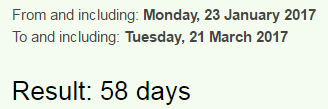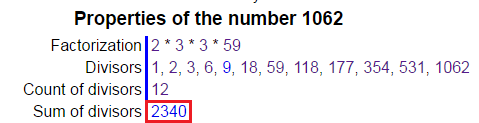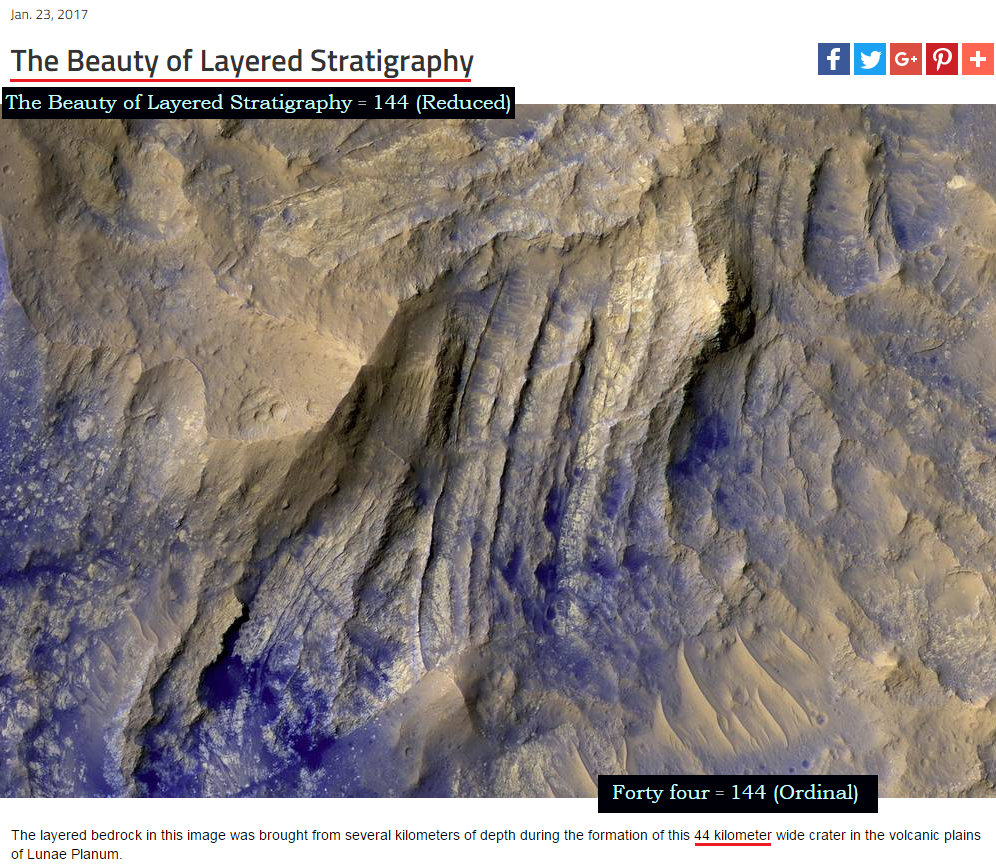CipherJan 23, 2017

Here's Looking at You, Photoshopped Tethys - beautiful codingHere's looking at you, Tethys = 8+5+9+5+1+3+6+6+11+9+5+7+1+2+7+6+3+2+5+2+8+7+1 = 119 (K/V Exception)
God Saturn = 7+15+4+19+1+20+21+18+14 = 119 (Ordinal)

It's clear as day why this post was released on 1/23/2017
Tethys = 46+5+20+8+25+19 = 123 (Francis Bacon)It was 'discovered' on 3/21/1684 - 3+2+1+1+6+8+4 = 25
Note that it was found in the time of Aries

Aries = 1+9+9+5+1 = 25 (Reduced)
Tethys = 2+5+2+8+7+1 = 25 (Reduced)

Giovanni Cassini died on 9/14/1712 - 9+1+4+1+7+1+2 = 25

Twenty first of March = 2+5+5+5+2+7+6+9+9+1+2+6+6+4+1+9+3+8 = 90 (Reduced)
Ninety = 5+9+5+5+2+7 = 33 (Reduced)
Tethys = 7+5+7+6+2+6 = 33 (Septenary)

This moon of Saturn was discovered by a Jesuit - Giovanni Cassini
Giovanni Cassini = 7+9+6+4+1+5+5+9+3+1+10+10+9+5+9 = 93 (S Exception)
Saturn = 19+1+20+21+18+14 = 93 (Ordinal)
On 3/21/2017 will be 333rd anniversary of the discovery of Tethys and it comes 58 days from 1/23/2017Saturn = 90+1+100+200+80+40 = 511 (Jewish)
Fifty-eight = 6+9+6+20+25+5+9+7+8+20 = 115 (Ordinal)
Cassini Probe = 3+1+1+1+9+5+9+7+9+6+2+5 = 58 (Reduced)
Discovered by 'G.D Cassini' = 7+4+3+1+10+10+9+5+9 = 58 (S Exception)
From anniversary of Cassini's death on 9/14/2017 to 333rd anniversary of discovery of Tethys is 33 weeks, 3 days234+432 = 666

Of course 1/23/2017 and 3/21/2017 have the same exact date numerology of 34 which is interesting
Tethys = 2+5+2+8+7+10 = 34 (S Exception)

Also I should mention that Tethys was found on 80th day in the year, 80 days before Cassini's birthdayEighty = 5+9+7+8+20+25 = 74 (Ordinal)
In NASA's post from today they say that the picture was taken on 11/10/2016 and coincidentally it took them 74 days to release it?
Tenth of November = 2+5+5+2+8+6+6+5+6+4+5+4+2+5+9 = 74 (Reduced)The picture was taken by Cassini spacecraft
Cassini = 3+1+19+19+9+14+9 = 74 (Ordinal)In the post they mention mountainous peak called "Sheria Montes" which has perfect gematria match with Saturn
Scheria Montes = 90+3+8+5+80+9+1+30+50+40+100+5+90 = 511 (Jewish)
Saturn = 90+1+100+200+80+40 = 511 (Jewish)
So let's go over the numbers they give us
They say that Tethys is 660 miles across and the picture was taken from 228,000 miles away?

There's something interesting with 660 and 228
660+606+066 = 1332
228+282+822 = 1332

six hundred sixty = 19+9+24+8+21+14+4+18+5+4+19+9+24+20+25 = 223 (Ordinal)
Masonic = 30+1+90+50+40+9+3 = 223 (Jewish)
The Synagogue of Satan = 223 (Ordinal)
Number of Skull and bones is a reflection of 223432+234 = 666Also 660 miles is 1062 kilometers as they say so at least they got something right, but there's a nice 1062 connection as wellOctal of 432 is 660

228,000 miles is 367,000 kilometers367 is 73rd prime number
21st prime number is 73 (Saturn = 21)
Seventy-three = 1+5+4+5+5+2+7+2+8+9+5+5 = 58 (Reduced)
Science = 58, Zodiac = 58,Freemasonry = 58, Secret Society = 58, Solar system = 58, Global Economy = 58

NASA, ESA, Italian Space Agency and JPL are all working (lying) together on this Cassini mission
European Space Agency = 5+200+80+50+60+5+1+40+90+60+1+3+5+1+7+5+40+3+400 = 1056 (Jewish)
156th prime number is 911
Jet Propulsion Laboratory = 1+5+2+7+9+6+7+3+3+10+9+6+5+3+1+2+6+9+1+2+6+9+7 = 119 (S Exception)
Saturn = 45+1+20+21+18+14 = 119 (Francis Bacon)
Star of David = 19+20+1+18+15+6+4+1+22+9+4 = 119 (Ordinal)
About an hour ago NASA released a hilarious post as always - here's the link https://www.nasa.gov/image-feature/jpl/pia12178/the-beauty-of-layered-stratigraphyNASA you might wanna change the way you code stuff.....such weak coding, step up your game please